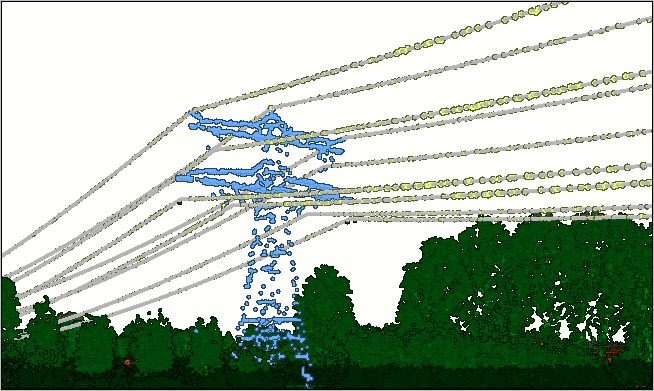# Extract Power Lines From Point Cloud (3D Analyst)

## Summary

Extracts 3D line features modeling power lines from classified point cloud data.

## Illustration## Usage

• This tool models each segment of a power line between two suspension points as individual line features. Points that belong to the same span are fitted to a catenary curve to determine the resulting line. The input point cloud must have the points representing the power lines classified with a unique class code value that excludes points representing other objects. The best results will be obtained with power line points that are regularly sampled at a distance that is at least half the length of the distance that separates the nearest cables.

• A span of bundled conductor cables, in which two or more conductor cables are affixed to each other, are best modeled as one line feature by specifying a point tolerance that approximates the radius of the bundled cables.

• Wind correction will only be applied in single direction. Consequently, the tool will not model power lines impacted by galloping vibrations that cause the power line to be displaced in diverging directions. Consider increasing the point tolerance if a desirable result is not produced for such power lines.

• The output line features will contain the following attributes:

• CLASS_CODE—The class code value associated with the power line.
• CURVE_LEN—The length of the catenary curve being modeled by the line. This value will differ from the 3D length of the line feature based on the value of the Output Line Tolerance parameter, where a smaller value will result in a more accurate depiction of the catenary curve than a larger value.
• WIND_ANGLE—The angle of deviation from the normal state when the power line is modeled with wind correction. A value of -1 is returned if no wind correction was attempted due to the distance specified in the Minimum Span For Wind Correction parameter.

## Syntax

`arcpy.3d.ExtractPowerLinesFromPointCloud(in_point_cloud, class_codes, out_3d_lines, {point_tolerance}, {separation_distance}, {max_sampling_gap}, {line_tolerance}, {wind_correction}, {min_wind_span}, {max_wind_deviation}, {end_point_search_radius}, {min_length})`
 Parameter Explanation Data Type in_point_cloud The LAS dataset layer containing points classified as power lines. LAS Dataset Layer class_codes[class_codes,...] The class code values for the points representing the power lines. Long out_3d_lines The 3D lines modeling the power lines. Feature Class point_tolerance(Optional) The distance used to establish the points that belong to a given power line. The default is 80 centimeters. Linear Unit separation_distance(Optional) The distance points must be to determine if they belong to different power lines. The default is 1 meter. Linear Unit max_sampling_gap(Optional) The largest gap that can exist in a given span of a power line. The catenary curve being modeled from a set of power line points will be extended by this distance to find other points that fit the same power line. The default is 5 meters. Linear Unit line_tolerance(Optional) The distance used to establish the accuracy of the output power line. A larger distance will result in the creation of less vertices per line, yielding a more coarse representation of the power line when compared with a smaller distance. The default is 1 centimeter. Linear Unit wind_correction(Optional) Specifies whether wind correction will be used to improve how points fit a given power line. Wind correction will only be applied in one direction and only for power lines with spans that are longer than the distance specified in the min_wind_span parameter. WIND —Wind correction will be used to improve the catenary curve's fitting of points belonging to the same power line. This is the default. NO_WIND — Wind correction will not be used, resulting in the creation of power lines that may not fit the points captured in the point cloud survey. Boolean min_wind_span(Optional) The shortest distance a power line span can be to apply wind correction when generating the output power line. The default is 60 meters. Linear Unit max_wind_deviation(Optional) The maximum angle that the wind is expected to deviate a given power line. The default is 10°. Double end_point_search_radius(Optional) The distance used to identify a common suspension point for power line segments connected to the same distribution pole or transmission tower. The default is 10 meters. Linear Unit min_length(Optional) The shortest wire length that can be used to determine the presence of a common end point. The default is 5 meters. Linear Unit

## Code sample

ExtractPowerLinesFromPointCloud example 1 (Python window)

The following sample demonstrates the use of this tool in the Python window.

``````import arcpy
arcpy.env.workspace = r'c:\data"
arcpy.ddd.ExtractPowerLinesFromPointCloud("Electrical_Assets.lasd", 14,
"Power_Lines.shp", "80 Centimeters")``````

## Licensing information

• Basic: Requires 3D Analyst
• Standard: Requires 3D Analyst